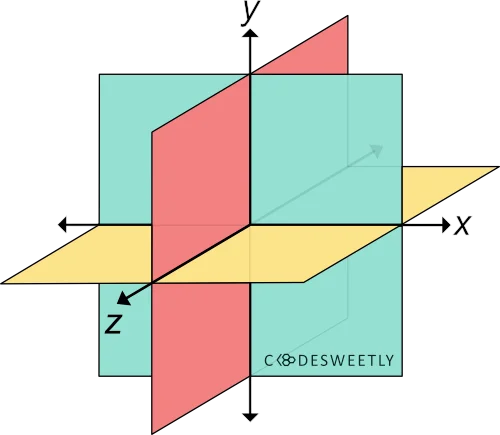# CSS scaleZ() Function – How to Resize Elements along Z-axis

scaleZ() transforms an element by resizing (scaling) it three-dimensionally from a fixed point along the z-axis.A three-dimensional Cartesian coordinate system showing the X-, Y-, and Z-axis
note
• "Transform origin" is the fixed point from which the computer scales an element.
• You can define your element's fixed point using the CSS transform-origin property. But the default is center.

## Syntax of the CSS scaleZ() Function​

scaleZ() accepts a single argument. Here is the syntax:

element {  transform: scaleZ(number);}

Note the following:

• The scaleZ(number) function is equivalent to scale3d(1, 1, number).
• The number argument specifies the element's scaling factor along the z-axis.
• Whenever you include scaling or zooming animations in your app, provide users an option to turn off animations. This option is necessary because scaling and zooming animations cause accessibility issues.

## Examples of the CSS scaleZ() Function​

We often use scaleZ() with other CSS functions such as perspective(), translateZ(), and rotateX(). Below are some examples.

### How to use scaleZ() with CSS perspective() and rotateX() functions​

img {  transform: perspective(370px) scaleZ(5) rotateX(17deg);  width: 80%;}

Try Editing It

Here's what we did in the snippet above:

1. We used the perspective() function to define a 370px distance between the user and the z=0 plane.
2. The scaleZ() function specifies a scale factor of 5 for the image along the z-axis.
3. We used the rotateX() function to rotate the image seventeen-degree (17⁰) around the x-axis.
info
• List perspective() first whenever you chain it with other CSS transform functions. Otherwise, browsers might transform the selected element incorrectly.
• List the scaleZ() function before rotateX(). Otherwise, the browser will not scale the element.

### How to use scaleZ() with CSS perspective() and translateZ() functions​

img {  width: 40%;}.second-image {  transform: perspective(370px) scaleZ(5) translateZ(30px);}

Try Editing It

Here's what we did in the snippet above:

1. We used the perspective() function to define a 370px distance between the user and the z=0 plane.
2. The scaleZ() function specifies a scale factor of 5 for the image along the z-axis.
3. We used the translateZ() function to reposition the second-image thirty pixels (30px) away from its original position along the z-axis.
info
• List perspective() first whenever you chain it with other CSS transform functions. Otherwise, browsers might transform the selected element incorrectly.
• List the scaleZ() function before translateZ(). Otherwise, the browser will not scale the element.

## Overview​

This article discussed what a CSS scaleZ() function is. We also used examples to see how it works.